# 1759 Pie Over Two

### Today’s Puzzle:

Today in the United States many students will celebrate pi day by eating pie. Today’s puzzle looks a little like a pie that has been cut in half, so I’m calling it pie over two, abbreviated as “π/2”.

Write the numbers 1 to 12 in the first column and again in the top row so that those numbers and the given clues make a multiplication table. Be sure to use logic every step of the way.### Factors of 1759:

• 1759 is a prime number.
• Prime factorization: 1759 is prime.
• 1759 has no exponents greater than 1 in its prime factorization, so √1759 cannot be simplified.
• The exponent in the prime factorization is 1. Adding one to that exponent we get (1 + 1) = 2. Therefore 1759 has exactly 2 factors.
• The factors of 1759 are outlined with their factor pair partners in the graphic below.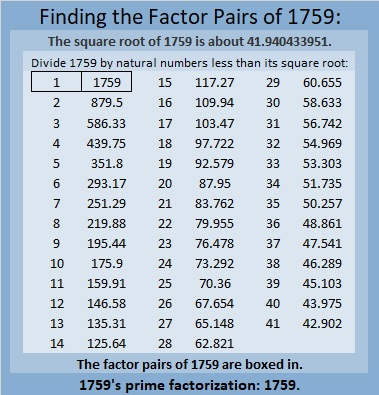How do we know that 1759 is a prime number? If 1759 were not a prime number, then it would be divisible by at least one prime number less than or equal to √1759. Since 1759 cannot be divided evenly by 2, 3, 5, 7, 11, 13, 17, 19, 23, 29, 31, 37, or 41, we know that 1759 is a prime number.

### More About the Number 1759:

Like every other odd number, 1759 is the difference of two squares:
880² – 879² = 1759.

OEIS.org informs us that 1759 is only the 17th Eisenstein-Mersenne prime number.

# 1745 How Thorny Is This Rose?

### Today’s Puzzle:

How thorny is this Valentine’s rose? That depends on if you use logic to solve it! It is a Level 5 puzzle, so some of the clues are meant to be tricky. Nevertheless, you can solve it! Just place all the numbers 1 to 10 in both the first column and the top row so that the given clues are the products of the numbers you write. There is only one solution.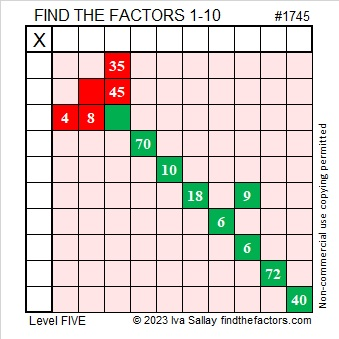Here’s the same puzzle minus the color: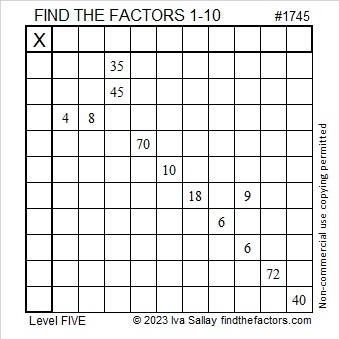### Factors of 1745:

• 1745 is a composite number.
• Prime factorization: 1745 = 5 × 349.
• 1745 has no exponents greater than 1 in its prime factorization, so √1745 cannot be simplified.
• The exponents in the prime factorization are 1 and 1. Adding one to each exponent and multiplying we get (1 + 1)(1 + 1) = 2 × 2 = 4. Therefore 1745 has exactly 4 factors.
• The factors of 1745 are outlined with their factor pair partners in the graphic below.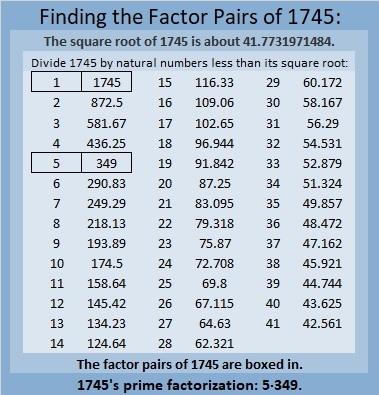### More About the Number 1745:

1745 is the difference of two squares in two different ways:
873² – 872² = 1745, and
177² – 172² = 1745.

1745 is also the sum of two squares in two different ways:
41² + 8² = 1745, and
31² + 28² = 1745.

1745 is the hypotenuse of FOUR Pythagorean triples:
177-1736-1745, calculated from 31² – 28², 2(31)(28), 31² + 28²,
656-1617-1745, calculated from 2(41)(8), 41² – 8², 41² + 8²,
900-1495-1745, which is 5 times (180-299-349)
1047-1396-1745, which is (3-4-5) times 349.

# 1735 What Amazing Mathematical Pattern I Noticed Today!

### Today’s Puzzle:

Will the common factor of 60 and 10 be 5 or will it be 10? Don’t guess. There is a better place to start this puzzle. After using logic to find some of the other factors, you will know if you should use 5 or 10. Don’t let me trip you up!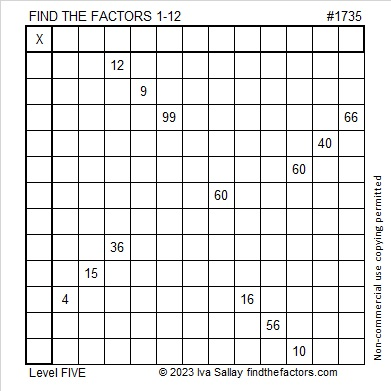### Factors of 1735:

• 1735 is a composite number.
• Prime factorization: 1735 = 5 × 347.
• 1735 has no exponents greater than 1 in its prime factorization, so √1735 cannot be simplified.
• The exponents in the prime factorization are 1 and 1. Adding one to each exponent and multiplying we get (1 + 1)(1 + 1) = 2 × 2 = 4. Therefore 1735 has exactly 4 factors.
• The factors of 1735 are outlined with their factor pair partners in the graphic below.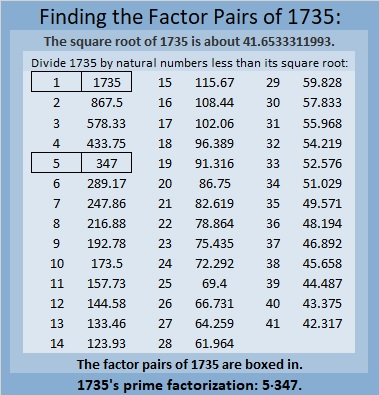### More About the Number 1735 and an Amazing Pattern:

1735 is the hypotenuse of a Pythagorean triple:
1041-1388-1735, which is (3-4-5) times 347.

1735 is 567 in base 18 because
5(18²) + 6(18) + 7(1) = 1735.

I know neither of those facts is amazing, but read on:

1735 is the sum of two, five, and ten consecutive positive numbers:
867 + 868 = 1735,
345 + 346 + 347 + 348 + 349 = 1735, and
169 + 170 + 171 + 172 + 173 + 174 + 175 + 176 + 177 + 178 = 1735.

1735 is the difference of two squares in two different ways:
868² – 867² = 1735, and
176² – 171² = 1735.

That first difference of two squares used the two consecutive numbers I listed above in red. Since mathematics is the study of patterns, I wondered if there would also be a relationship of squares with the ten consecutive numbers in blue. I typed into my calculator:
178² – 177² + 176² – 175² + 174² – 173² + 172² – 171² + 170² – 169² =

Guess what the answer was? If you guessed 1735, you would be right! Oh, mathematics is certainly filled with amazing patterns! I’m thrilled that I spotted this one! It appears something similar can be written whenever a number is the sum of an even amount of consecutive numbers! I find that amazing!

I also noticed that the second difference of two squares noted above is contained in the string. I underlined those two squares. Since the difference of those squares by themselves equals the whole string, it follows that the rest of those squares added and subtracted equal zero. That means the sum of the rest of the positive terms must equal the sum of the rest of the negative terms:
178² + 174² + 172² + 170² = 120144 = 177² + 175² + 173² + 169².
I’ve never found a string of four squares equal to another string of four squares before, so naturally, I’m excited! I’m anxious to apply this pattern to other numbers that are the sum of ten consecutive numbers. For example, the first ten numbers add up to 55.
10² – 9² + – 7² + 6² – 5² + 4² – 3² + 2² – 1² =55. And since 8² – 3² = 55, it follows that
10²  + 6² + 4² + 2² = 156 = 9² + 7² + 5²+ 1².
It is no longer “impossible” for me to find these equal sums! Here’s another that connects this year with other recent years:We can all hope this equation doesn’t predict the future. Ha ha! I’m confident it is NOT a bad omen!

# 1724 Carol of the Ukrainian Bells

### Today’s Puzzle:

As I sit in my warm, peaceful house, I often think about the people of Ukraine whose country has been ravaged by war. Many of them, including children, are also facing a winter with no heat. My heart goes out to them.

My childhood was so unlike theirs. I was able to attend school classes and learn about many different topics without fear of dying. In Junior High School choir class, one of the songs I learned was called Carol of the Bells. I just recently learned from Slate magazine of the song’s Ukrainian roots:

A little over a hundred years ago Mykola Leontovych, a Ukrainian composer, arranged several of his country’s folk songs together in a piece he titled Shchedryk. Tragically, he was murdered by a Russian assassin on January 23, 1921, in the Red Terror, when the Bolsheviks were intent on eliminating Ukrainian leaders, intellectuals, and clergy.

During this time of great unrest, the Ukrainian National Chorus performed Shchedryk around the world and in cities large and small in the United States. One performance was even given at the famed Carnegie Hall on October 5, 1922. The haunting melody was heard by Peter Wilhousky who penned alternate words for it: Hark how the bells, Sweet silver bells,…

Now it is one of our most beloved Christmas carols. I am grateful I learned the words and tune in junior high, although I wish I had learned of its Ukrainian history then as well.

These two bells puzzles are reminiscent of Ukraine’s flag. Long may it wave. Write each number 1 to 10 in the yellow columns and rows so that the given clues are the products of the numbers you write.Here are the same puzzles if you prefer to use less of your printer ink.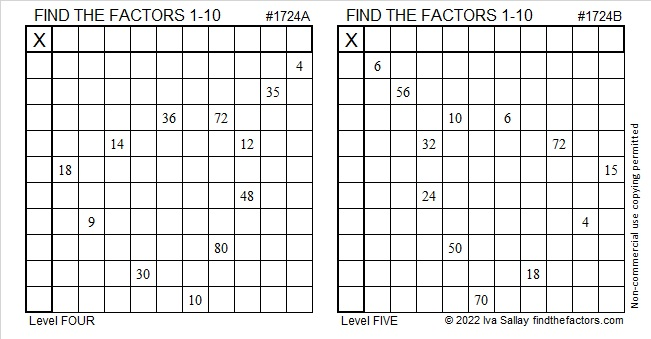### Factors of 1724:

• 1724 is a composite number.
• Prime factorization: 1724 = 2 × 2 × 431, which can be written 1724 = 2² × 431.
• 1724 has at least one exponent greater than 1 in its prime factorization so √1724 can be simplified. Taking the factor pair from the factor pair table below with the largest square number factor, we get √1724 = (√4)(√431) = 2√431.
• The exponents in the prime factorization are 2 and 1. Adding one to each exponent and multiplying we get (2 + 1)(1 + 1) = 3 × 2 = 6. Therefore 1724 has exactly 6 factors.
• The factors of 1724 are outlined with their factor pair partners in the graphic below.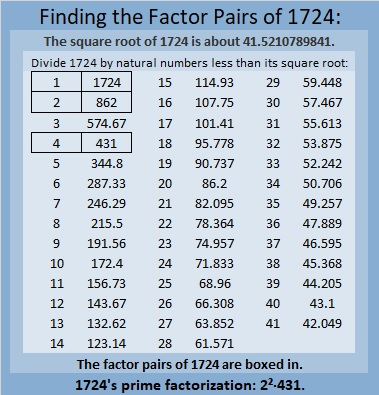### More About the Number 1724:

1724 is the difference of two squares:
432² – 430² = 1724.

1724₁₀ = 464₂₀, a palindrome, because
4(20²) + 6(20¹) + 4(20°) = 1724.

# 1688 What Can Grow from a Little Seed?

### Today’s Puzzle:

Pumpkins are often harvested this time of year. It is amazing how one little seed properly planted and tended can grow into a vine that produces pumpkin after pumpkin after pumpkin.  Indeed great things can come from little things.

This level 5 puzzle starts out fairly easy. It doesn’t get very complicated until you’re about halfway through. Don’t give up! Your mind will grow as you use logic to find a way to work it out. Write the numbers from 1 to 12 in both the first column and the top row so that those numbers and the given clues make a multiplication table.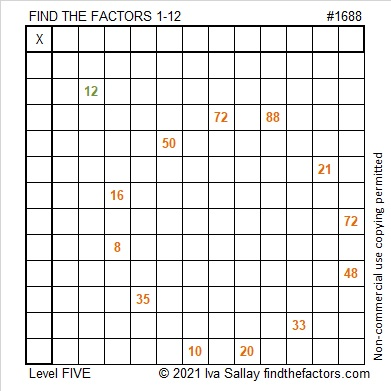### Factors of 1688:

• 1688 is a composite number.
• Prime factorization: 1688 = 2 × 2 × 2 × 211, which can be written 1688 = 2³ × 211.
• 1688 has at least one exponent greater than 1 in its prime factorization so √1688 can be simplified. Taking the factor pair from the factor pair table below with the largest square number factor, we get √1688 = (√4)(√422) = 2√422.
• The exponents in the prime factorization are 3 and 1. Adding one to each exponent and multiplying we get (3 + 1)(1 + 1) = 4 × 2 = 8. Therefore 1688 has exactly 8 factors.
• The factors of 1688 are outlined with their factor pairs in the graphic below.### More About the Number 1688:

1688 is the difference of two squares in two different ways:
423² – 421² = 1688, and
213² – 209² = 1688.

1688₁₀ = 888₁₄ because 8(14² + 14¹ + 14º) = 1688.

# 1678 and Level 5

### Today’s Puzzle:

Using logic, write the numbers from 1 to 10 in both the first column and the top row so that this puzzle will function like a multiplication table.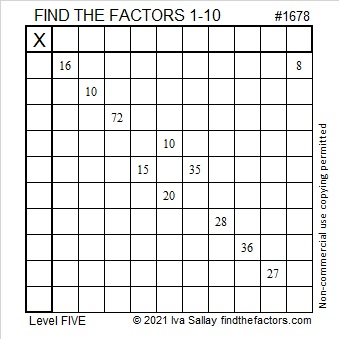### Factors of 1678:

• 1678 is a composite number.
• Prime factorization: 1678 = 2 × 839.
• 1678 has no exponents greater than 1 in its prime factorization, so √1678 cannot be simplified.
• The exponents in the prime factorization are 1 and 1. Adding one to each exponent and multiplying we get (1 + 1)(1 + 1) = 2 × 2 = 4. Therefore 1678 has exactly 4 factors.
• The factors of 1678 are outlined with their factor pair partners in the graphic below.### More about the number 1678:

Because 2(839)(1) = 1678, you can calculate the only Pythagorean triple that contains the number 1678:
The smaller leg will be 2(839)(1) = 1678.
The longer leg will be 839² – 1² = 703920.
The hypotenuse will be 839² + 1² = 703922.

# 1668 and Level 5

### Today’s Puzzle:

Write the numbers 1 to 12 in both the first column and the top row so that those numbers and the given clues create a multiplication table. This is a level 5 puzzle, so some of the clues may be tricky. Remember to use logic and consider all possibilities for factors to avoid being tricked.### Factors of 1668:

• 1668 is a composite number.
• Prime factorization: 1668 = 2 × 2 × 3 × 139, which can be written 1668 = 2² × 3 × 139.
• 1668 has at least one exponent greater than 1 in its prime factorization so √1668 can be simplified. Taking the factor pair from the factor pair table below with the largest square number factor, we get √1668 = (√4)(√417) = 2√417.
• The exponents in the prime factorization are 2, 1, and 1. Adding one to each exponent and multiplying we get (2 + 1)(1 + 1)(1 + 1) = 3 × 2 × 2 = 12. Therefore 1668 has exactly 12 factors.
• The factors of 1668 are outlined with their factor pair partners in the graphic below.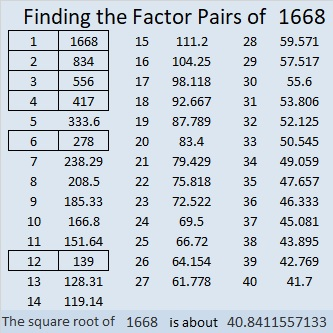### More About the Number 1668:

1668 is the difference of two squares in two different ways:
418² – 416² = 1668, and
142² – 136² = 1668.

# 1655 and Level 5

### Today’s Puzzle:

Using logic, write all the numbers from 1 to 10 in both the first column and the top row of this puzzle so that those numbers are the factors of the given clues.### Factors of 1655:

• 1655 is a composite number.
• Prime factorization: 1655 = 5 × 331.
• 1655 has no exponents greater than 1 in its prime factorization, so √1655 cannot be simplified.
• The exponents in the prime factorization are 1 and 1. Adding one to each exponent and multiplying we get (1 + 1)(1 + 1) = 2 × 2 = 4. Therefore 1655 has exactly 4 factors.
• The factors of 1655 are outlined with their factor pair partners in the graphic below.### More About the Number 1655:

1655 is the hypotenuse of a Pythagorean triple:
993-1324-1655, which is (3-4-5) times 331.

# 1644 Level 5 Puzzles Are Not So Easy to Solve

### Today’s Puzzle:

This puzzle isn’t so easy to solve. For example, the common factor of clues 48 and 24 might be 4, 6, 8, or 12. Which one should you use? Logic will help answer that question. Give this puzzle a try?### Factors of 1644:

1644 has 12 factors and is divisible by 12.

• 1644 is a composite number.
• Prime factorization: 1644 = 2 × 2 × 3 × 137, which can be written 1644 = 2² × 3 × 137.
• 1644 has at least one exponent greater than 1 in its prime factorization so √1644 can be simplified. Taking the factor pair from the factor pair table below with the largest square number factor, we get √1644 = (√4)(√411) = 2√411.
• The exponents in the prime factorization are 2, 1, and 1. Adding one to each exponent and multiplying we get (2 + 1)(1 + 1)(1 + 1) = 3 × 2 × 2 = 12. Therefore 1644 has exactly 12 factors.
• The factors of 1644 are outlined with their factor pair partners in the graphic below.### More about the Number 1644:

1644 is the hypotenuse of a Pythagorean triple:
1056-1260-1644 which is 12 times (88-105-137).

# 1633 and Level 5

### Today’s Puzzle:

It might be tricky in a few places, but use logic to write the numbers from 1 to 10 in both the first column and the top row so that those numbers and the given clues behave like a multiplication table.### Factors of 1633:

• 1633 is a composite number.
• Prime factorization: 1633 = 23 × 71.
• 1633 has no exponents greater than 1 in its prime factorization, so √1633 cannot be simplified.
• The exponents in the prime factorization are 1 and 1. Adding one to each exponent and multiplying we get (1 + 1)(1 + 1) = 2 × 2 = 4. Therefore 1633 has exactly 4 factors.
• The factors of 1633 are outlined with their factor pair partners in the graphic below.### More about the Number 1633:

1633 is the difference of two squares in two different ways:
817² – 816² = 1633, and
47² – 24² = 1633.